# 面试官本拿求素数搞我，但被我优雅的“回击“了(素数筛)

## 求一个质数

boolean isprime(int value){
for(int i=2;i<value;i++)
{
if(value%i==0)
{return false;}
}
return true;
}


boolean isprime(int value)
{
for(int i=2;i*i<value+1;i++)
{
if(value%i==0)
{return false;}
}
return true;
}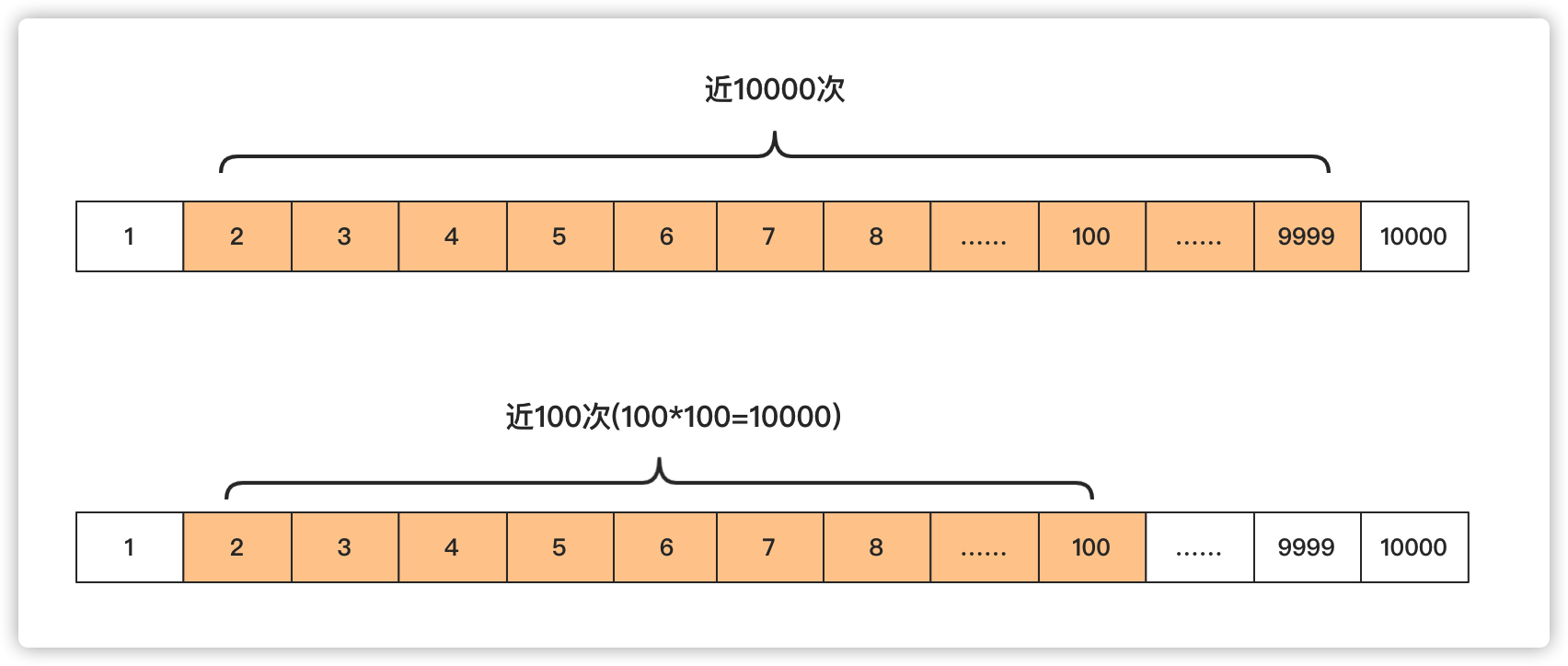## 求多个素数

### 埃拉托斯特尼(Eratosthenes)筛法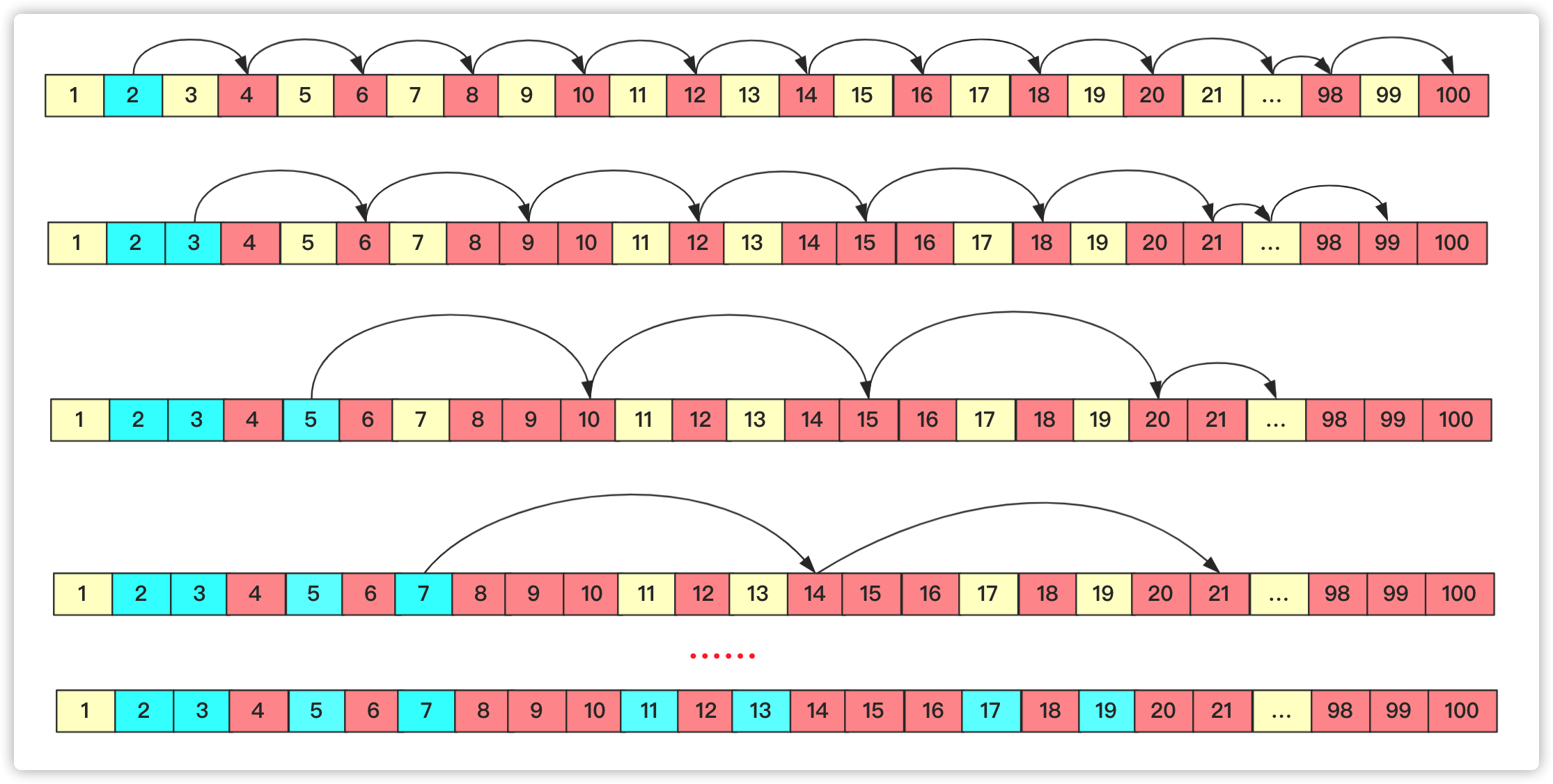boolean isprime[];
long prime[];
void getprime()
{
prime=new long;//记录第几个prime
int index=0;//标记prime当前下标
isprime=new boolean ;//判断是否被标记过
for(int i=2;i<1000001;i++)
{
if(!isprime[i])
{
prime[index++]=i;
}
for(int j=i+i;j<1000000;j=j+i)//他的所有倍数都over
{
isprime[j]=true;
}
}
}



## 欧拉筛

• 在遍历到一个数的时候，如果这个数没被标记，那么这个数存在素数的数组中，对应下标加1.
• 不管这个数是不是素数，遍历已知素数将它和该素数的乘积值标记，如果这个素数能够被当前值i整除，那么停止操作进行下一轮。

boolean isprime[];
int prime[];
void getprimeoula()// 欧拉筛
{
prime = new int;// 记录第几个prime
int index = 0;
isprime = new boolean;
for (int i = 2; i < 1000001; i++) {
if (!isprime[i]) {
prime[index++] = i;
}
for (int j = 0; j < index && i * prime[j] <= 100000; j++){//已知素数范围内枚举
isprime[i * prime[j]] = true;// 标记乘积
if (i % prime[j] == 0)
break;
}
}
}


i*prime[j+1]=(prime[j]*k)*prime[j+1]=prime[j]*(k*prime[j+1])i=k*prime[j+1]两个位置就产生冲突重复计算啦,所以一旦遇到能够被整除的就停止。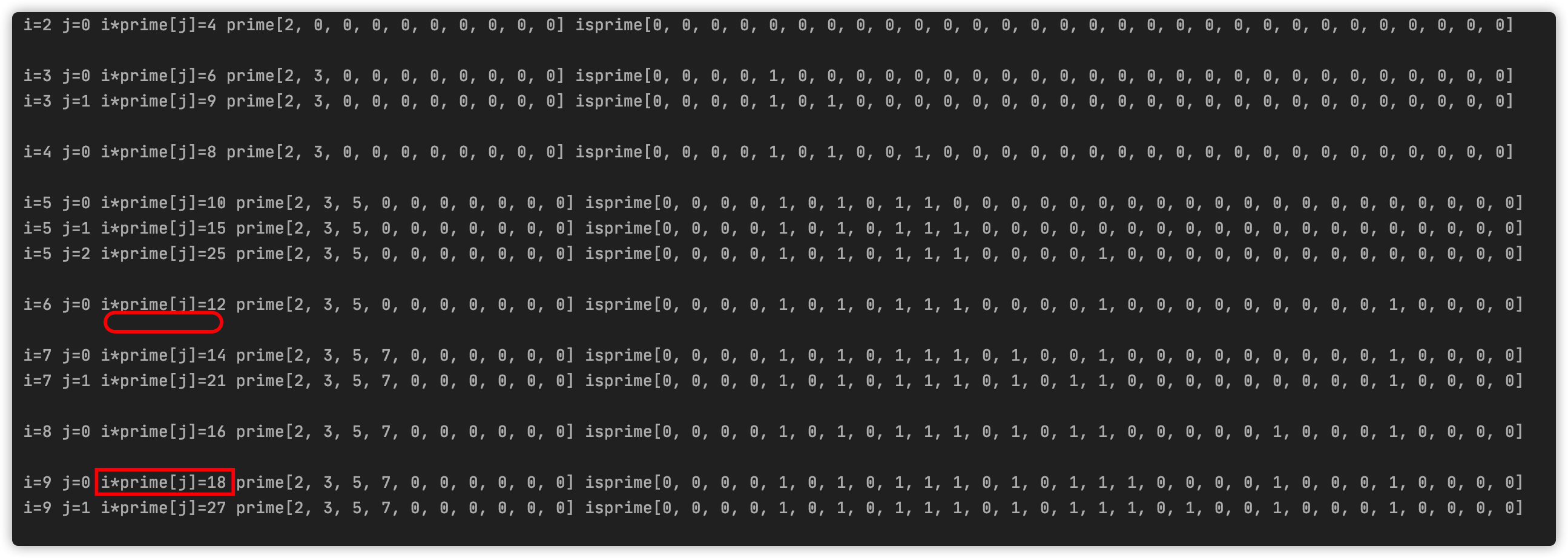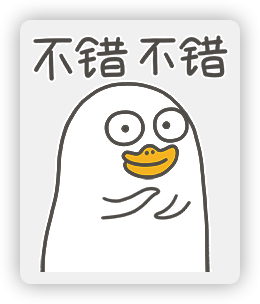1. 点赞支持一下， 您的肯定是我在csdn创作的源源动力。

2. 微信搜索「bigsai」，关注我的公众号(新人求支持)，不仅免费送你电子书，我还会第一时间在公众号分享知识技术。加我还可拉你进力扣打卡群一起打卡LeetCode。Big sai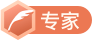CSDN认证博客专家 数据结构与算法 爬虫 Java

10-21198610-161258
05-081118
10-182万+
11-272473
11-138749
04-276865
10-273万+
03-222479
07-25824
02-12689
05-291万+
02-201745
03-101019
12-101万+
03-26698
09-05600
09-242484
03-225703点击重新获取扫码支付余额充值